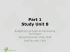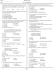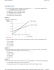# C1 prep set mock week

## Transcription

C1 prep set mock week
```Given y = x3 + 4x + 1, find the value of
1.
when x = 3.
(4) 2.
Find
– √27 in the form k√3, where k is an integer.
(4)
3.
Find
,
giving each term in its simplest form.
(4)
4.
The line L1 has equation 4x + 2y – 3 = 0.
(a) Find the gradient of L1.
(2)
The line L2 is perpendicular to L1 and passes through the point (2, 5).
(b) Find the equation of L2 in the form y = mx+ c, where m and c are constants.
5.
(3)
Solve
(a) 2y = 8,
(1)
(b) 2x × 4x + 1 = 8.
(4)
6.
A sequence x1, x2, x3, ... is defined by
x1 = 1,
xn +1 = (xn)2 – kxn,
n ≥ 1,
where k is a constant.
(a) Find an expression for x2 in terms of k.
(1)
(b) Show that x3 = 1 – 3k + 2k2.
(2)
Given also that x3 = 1,
(c) calculate the value of k.
(3)
(d) Hence find the value of
.
(3)
7.
Each year, Abbie pays into a savings scheme. In the first year she pays in £500. Her payments
then increase by £200 each year so that she pays £700 in the second year, £900 in the third year
and so on.
(a) Find out how much Abbie pays into the savings scheme in the tenth year.
(2)
Abbie pays into the scheme for n years until she has paid a total of £67 200.
(b) Show that n2 + 4n – 24 × 28 = 0.
(5)
(b) Hence find the number of years that Abbie pays into the savings scheme.
(2)
P42823A
3
Turn over
8.
A rectangular room has a width of x m.
The length of the room is 4 m longer than its width.
Given that the perimeter of the room is greater than 19.2 m,
(a) show that x > 2.8.
(3)
Given also that the area of the room is less than 21 m2,
(b) (i) write down an inequality, in terms of x, for the area of the room.
(ii) Solve this inequality.
(4)
(c) Hence find the range of possible values for x.
(1)
9.
Figure 1
Figure 1 shows a sketch of the curve C with equation y = f(x).
The curve C passes through the point (–1, 0) and touches the x-axis at the point (2, 0).
The curve C has a maximum at the point (0, 4).
The equation of the curve C can be written in the form.
y = x3 + ax2 + bx + c,
where a, b and c are integers.
(a) Calculate the values of a, b and c.
(5)
(b) Sketch the curve with equation y = f( x).
Show clearly the coordinates of all points where the curve crosses or meets the coordinate
axes.
(3)
P42823A
5
10.
A curve has equation y = f(x). The point P with coordinates (9, 0) lies on the curve.
Given that
f'(x) =
,
x > 0,
(a) find f(x).
(6)
(b) Find the x-coordinates of the two points on y = f(x) where the gradient of the curve is equal
to 10.
(4)
11.
Figure 2
The line y = x + 2 meets the curve x2 + 4y2 – 2x = 35 at the points A and B as shown in Figure 2.
(a) Find the coordinates of A and the coordinates of B.
(6)
(b) Find the distance AB in the form r√2, where r is a rational number.
(3)
TOTAL FOR PAPER: 75 MARKS
END
```

### Parabolic Curve### Paper G QP â C2 Solomon - Mathematics Christopher Page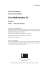### A 30.00 milliliter sample of a weak monoprotic acid was... solution of NaOH.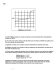### Juvenile Idiopathic Scoliosis Reference Guide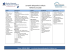### 3 sample exam paper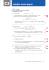### Production Manager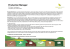### Economics Assignment - dav international school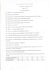### MAT 132 - Calculus II,Practice Problems for Midterm 2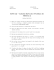### C2 Worksheet D - WordPress.com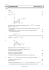### Economics 2301-03 SAMPLE QUESTIONS FOR MIDTERM EXAM #2 Fall, 2011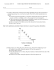### Math 1172 Sample Test #1 + 1 and the line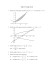### Ballasted R40 Curve Baseplates - L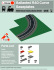### lesto - Dux Lighting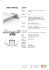### where the girls are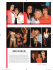### Long Term Ground Reaction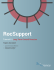### Supply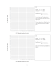### Assignment 1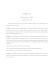### SN CURVE APPROACH FOR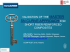### when to Fit_4lr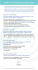### Study Unit 2 - CMAPrepCourse